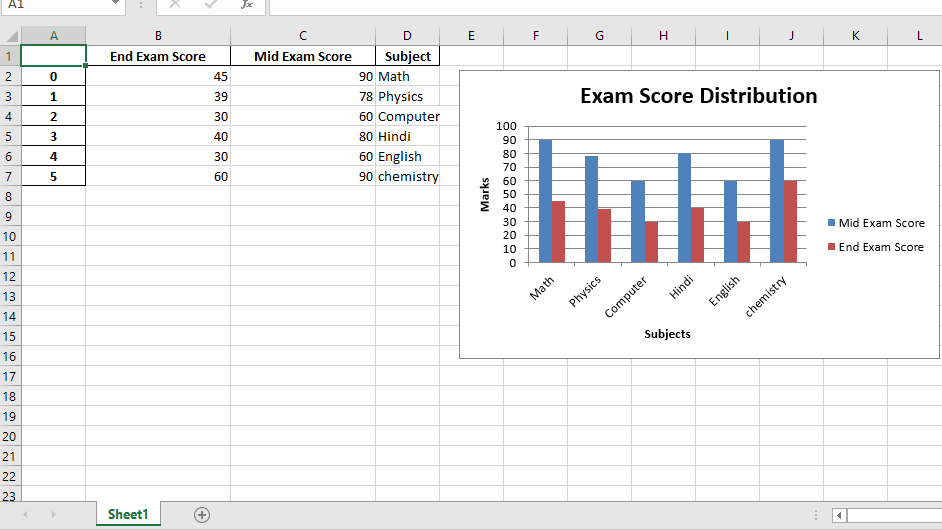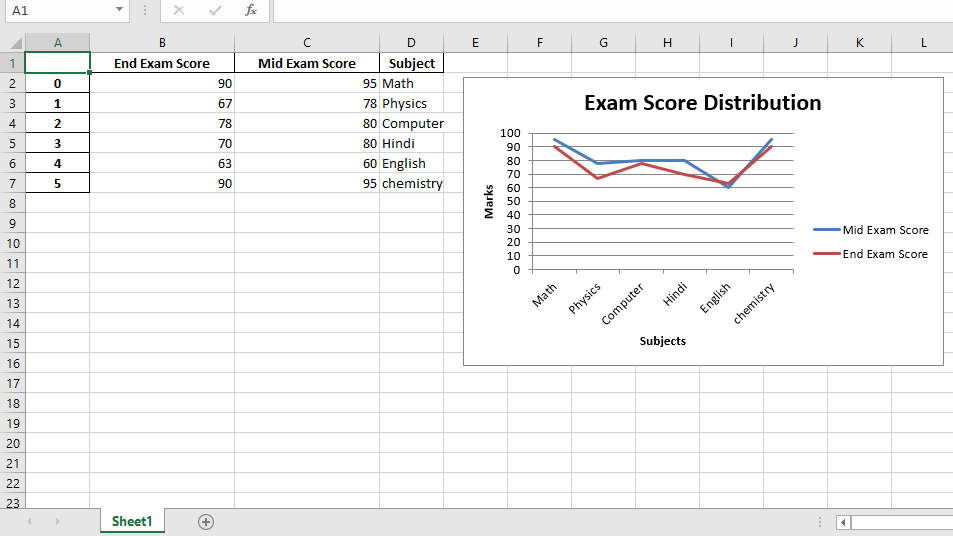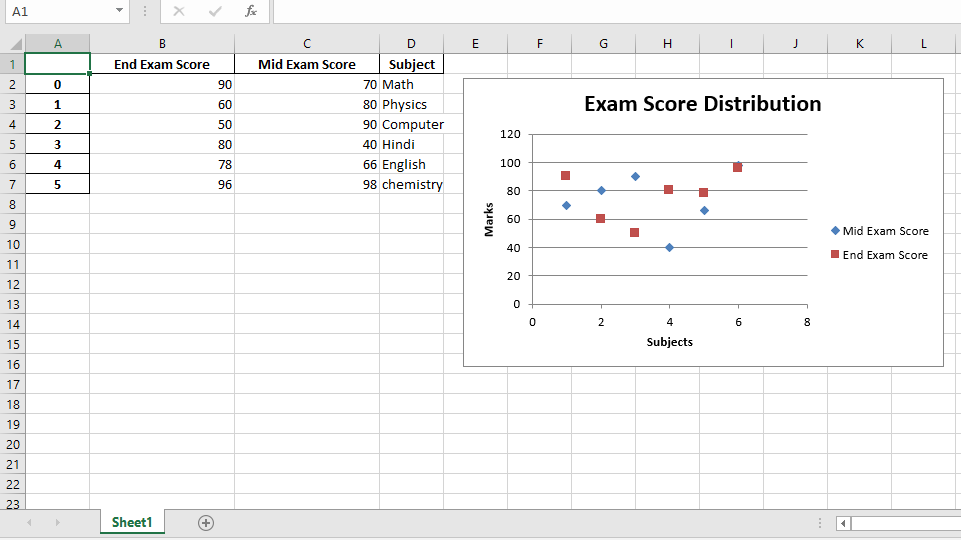Related Articles

# Python | Working with Pandas and XlsxWriter | Set – 3

• Difficulty Level : Hard
• Last Updated : 28 Dec, 2018

Prerequisite: : Python working with pandas and xlsxwriter | set-1

Python Pandas is a data analysis library. It can read, filter and re-arrange small and large datasets and output them in a range of formats including Excel.

Pandas writes Excel files using the XlsxWriter modules.

`XlsxWriter` is a Python module for writing files in the XLSX file format. It can be used to write text, numbers, and formulas to multiple worksheets. Also, it supports features such as formatting, images, charts, page setup, auto filters, conditional formatting and many others.

Code #1 : Plot a Column chart using Pandas and XlsxWriter.

 `# import pandas library as pd``import` `pandas as pd`` ` `# Create a Pandas dataframe from some data.``dataframe ``=` `pd.DataFrame({``                    ``'Subject'``: [``"Math"``, ``"Physics"``, ``"Computer"``,``                                ``"Hindi"``, ``"English"``, ``"chemistry"``],``                   ``'Mid Exam Score'` `:  [``90``, ``78``, ``60``, ``80``, ``60``, ``90``],``                   ``'End Exam Score'` `: [``45``, ``39``, ``30``, ``40``, ``30``, ``60``] })`` ` `# Create a Pandas Excel writer ``# object using XlsxWriter as the engine.``writer_object ``=` `pd.ExcelWriter(``'pandas_column_chart.xlsx'``,``                                     ``engine ``=``'xlsxwriter'``)`` ` `# Write a dataframe to the worksheet.``dataframe.to_excel(writer_object, sheet_name ``=``'Sheet1'``)`` ` `# Create xlsxwriter workbook object .``workbook_object ``=` `writer_object.book`` ` `# Create xlsxwriter worksheet object``worksheet_object ``=` `writer_object.sheets[``'Sheet1'``]`` ` `# set width of the B and C column``worksheet_object.set_column(``'B:C'``, ``20``)`` ` `# Create a chart object that can be added  ``# to a worksheet using add_chart() method.  ``   ` `# here we create a column chart object .``chart_object ``=` `workbook_object.add_chart({``'type'``: ``'column'``})`` ` `# Add a data series to a chart  ``# using add_series method.`` ` `# Configure the first series.  ``# syntax to define ranges is : ``# [sheetname, first_row, first_col, last_row, last_col].``chart_object.add_series({``    ``'name'``:       [``'Sheet1'``, ``0``, ``2``],  ``    ``'categories'``: [``'Sheet1'``, ``1``, ``3``, ``6``, ``3``],  ``    ``'values'``:     [``'Sheet1'``, ``1``, ``2``, ``6``, ``2``],  ``    ``})`` ` `# Configure a second series.``chart_object.add_series({``    ``'name'``:       [``'Sheet1'``, ``0``, ``1``],  ``    ``'categories'``: [``'Sheet1'``, ``1``, ``3``, ``6``, ``3``],  ``    ``'values'``:     [``'Sheet1'``, ``1``, ``1``, ``6``, ``1``],  ``    ``})`` ` `# Add a chart title.``chart_object.set_title({``'name'``: ``'Exam Score Distribution'``})`` ` `# Add x-axis label ``chart_object.set_x_axis({``'name'``: ``'Subjects'``}) ``   ` `# Add y-axis label ``chart_object.set_y_axis({``'name'``: ``'Marks'``})`` ` `# add chart to the worksheet with given``# offset values at the top-left corner of``# a chart is anchored to cell E2``worksheet_object.insert_chart(``'E2'``, chart_object, ``                ``{``'x_offset'``: ``20``, ``'y_offset'``: ``5``})`` ` `# Close the Pandas Excel writer ``# object and output the Excel file. ``writer_object.save()`

Output :Code #2 : Plot a Line chart using Pandas and XlsxWriter.

 `# import pandas library as pd``import` `pandas as pd`` ` `# Create a Pandas dataframe from some data.``dataframe ``=` `pd.DataFrame({``                    ``'Subject'``: [``"Math"``, ``"Physics"``, ``"Computer"``, ``                                ``"Hindi"``, ``"English"``, ``"chemistry"``],``                   ``'Mid Exam Score'` `:  [``95``, ``78``, ``80``, ``80``, ``60``, ``95``],``                   ``'End Exam Score'` `: [``90``, ``67``, ``78``, ``70``, ``63``, ``90``]``                    ``})`` ` `# Create a Pandas Excel writer ``# object using XlsxWriter as the engine.``writer_object ``=` `pd.ExcelWriter(``'pandas_line_chart.xlsx'``, ``                                   ``engine ``=``'xlsxwriter'``)`` ` `# Write a dataframe to the worksheet.``dataframe.to_excel(writer_object, sheet_name ``=``'Sheet1'``)`` ` `# Create xlsxwriter workbook object .``workbook_object ``=` `writer_object.book`` ` `# Create xlsxwriter worksheet object``worksheet_object ``=` `writer_object.sheets[``'Sheet1'``]`` ` `# set width of the B and C column``worksheet_object.set_column(``'B:C'``, ``20``)`` ` ` ` `# Create a chart object that can be added  ``# to a worksheet using add_chart() method.  ``   ` `# here we create a line chart object .``chart_object ``=` `workbook_object.add_chart({``'type'``: ``'line'``})`` ` `# Add a data series to a chart  ``# using add_series method.`` ` `# Configure the first series.  ``# syntax to define ranges is : ``# [sheetname, first_row, first_col, last_row, last_col].``chart_object.add_series({``    ``'name'``:       [``'Sheet1'``, ``0``, ``2``],  ``    ``'categories'``: [``'Sheet1'``, ``1``, ``3``, ``6``, ``3``],  ``    ``'values'``:     [``'Sheet1'``, ``1``, ``2``, ``6``, ``2``],  ``    ``})`` ` `# Configure a second series.``chart_object.add_series({``    ``'name'``:       [``'Sheet1'``, ``0``, ``1``],  ``    ``'categories'``: [``'Sheet1'``, ``1``, ``3``, ``6``, ``3``],  ``    ``'values'``:     [``'Sheet1'``, ``1``, ``1``, ``6``, ``1``],  ``    ``})`` ` `# Add a chart title.``chart_object.set_title({``'name'``: ``'Exam Score Distribution'``})`` ` `# Add x-axis label ``chart_object.set_x_axis({``'name'``: ``'Subjects'``}) ``   ` `# Add y-axis label ``chart_object.set_y_axis({``'name'``: ``'Marks'``})`` ` `# add chart to the worksheet with given``# offset values at the top-left corner of``# a chart is anchored to cell E2``worksheet_object.insert_chart(``'E2'``, chart_object, ``                 ``{``'x_offset'``: ``20``, ``'y_offset'``: ``5``})`` ` `# Close the Pandas Excel writer ``# object and output the Excel file. ``writer_object.save()`

Output :Code #3 : Plot a Scatter chart using Pandas and XlsxWriter.

 `# import pandas library as pd``import` `pandas as pd`` ` `# Create a Pandas dataframe from some data.``dataframe ``=` `pd.DataFrame({``                    ``'Subject'``: [``"Math"``, ``"Physics"``, ``"Computer"``,``                             ``"Hindi"``, ``"English"``, ``"chemistry"``],``                   ``'Mid Exam Score'` `:  [``70``, ``80``, ``90``, ``40``, ``66``, ``98``],``                   ``'End Exam Score'` `: [``90``, ``60``, ``50``, ``80``, ``78``, ``96``]``                    ``})`` ` `# Create a Pandas Excel writer ``# object using XlsxWriter as the engine.``writer_object ``=` `pd.ExcelWriter(``'pandas_Scatter_chart.xlsx'``,``                                      ``engine ``=``'xlsxwriter'``)`` ` `# Write a dataframe to the worksheet.``dataframe.to_excel(writer_object, sheet_name ``=``'Sheet1'``)`` ` `# Create xlsxwriter workbook object .``workbook_object ``=` `writer_object.book`` ` `# Create xlsxwriter worksheet object``worksheet_object ``=` `writer_object.sheets[``'Sheet1'``]`` ` `# set width of the B and C column``worksheet_object.set_column(``'B:C'``, ``20``)`` ` ` ` `# Create a chart object that can be added  ``# to a worksheet using add_chart() method.  ``   ` `# here we create a scatter chart object .``chart_object ``=` `workbook_object.add_chart({``'type'``: ``'scatter'``})`` ` `# Add a data series to a chart  ``# using add_series method.`` ` `# Configure the first series.  ``# syntax to define ranges is : ``# [sheetname, first_row, first_col, last_row, last_col].``chart_object.add_series({``    ``'name'``:       [``'Sheet1'``, ``0``, ``2``],  ``    ``'categories'``: [``'Sheet1'``, ``1``, ``3``, ``6``, ``3``],  ``    ``'values'``:     [``'Sheet1'``, ``1``, ``2``, ``6``, ``2``],  ``    ``})`` ` `# Configure a second series.``chart_object.add_series({``    ``'name'``:       [``'Sheet1'``, ``0``, ``1``],  ``    ``'categories'``: [``'Sheet1'``, ``1``, ``3``, ``6``, ``3``],  ``    ``'values'``:     [``'Sheet1'``, ``1``, ``1``, ``6``, ``1``],  ``    ``})`` ` `# Add a chart title.``chart_object.set_title({``'name'``: ``'Exam Score Distribution'``})`` ` `# Add x-axis label ``chart_object.set_x_axis({``'name'``: ``'Subjects'``}) ``   ` `# Add y-axis label ``chart_object.set_y_axis({``'name'``: ``'Marks'``})`` ` `# add chart to the worksheet with given``# offset values at the top-left corner of``# a chart is anchored to cell E2``worksheet_object.insert_chart(``'E2'``, chart_object, ``                ``{``'x_offset'``: ``20``, ``'y_offset'``: ``5``})`` ` `# Close the Pandas Excel writer ``# object and output the Excel file. ``writer_object.save()`

Output :Attention geek! Strengthen your foundations with the Python Programming Foundation Course and learn the basics.

To begin with, your interview preparations Enhance your Data Structures concepts with the Python DS Course. And to begin with your Machine Learning Journey, join the Machine Learning – Basic Level Course

My Personal Notes arrow_drop_up